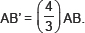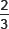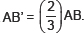# Constructions-Important Questions

Class IX Math
Hots for Construction
1.   Draw a circle of diameter 6.4 cm. Then draw two tangents to the circle from a point P at a distance 6.4 cm from the centre of the circle.
2.   Draw a circle of radius 3.4 cm. Draw two tangents to it inclinded at tn angle of 60° to each other:
3.   Draw ΔABC in which AB = 3.8 cm, ∠B = 60° and median AD = 3.6 cm. Draw another triangle AB’C similar to the first such that4.   Draw an equiliateral triangle of height 3.6 cm. Draw another triangle similar to it such that its side isof the side of the first.
5.   Draw an isosceles ΔABC, in which AB = AC = 5.6 cm and ∠ABC = 60°. Draw another ΔAB’C’ similar to ΔABC such that